# RD Sharma Solutions For Class 7 Maths Chapter 20 Mensuration - I (Perimeter and Area of Rectilinear Figures) Exercise 20.1

For students who wish to excel in their exams and build their confidence level, practising RD Sharma Class 7 textbook is essential. Students can download the PDF of RD Sharma Solutions for Class 7 Exercise 20.1 of Chapter 20 Mensuration – I (Perimeter and Area of Rectilinear Figures) from the links provided below. This exercise deals with perimeter and area of squares and rectangles. Some of the topics of this exercise are listed below.

• Units of measurement of area
• Square milimeter
• Square centimeter
• Square decimeter
• Square meter
• Hectare
• Square kilometer
• Perimeter and area of squares and rectangles

## Download the PDF of RD Sharma Solutions For Class 7 Maths Chapter 20 – Mensuration – I (Perimeter and Area of Rectilinear Figures) Exercise 20.1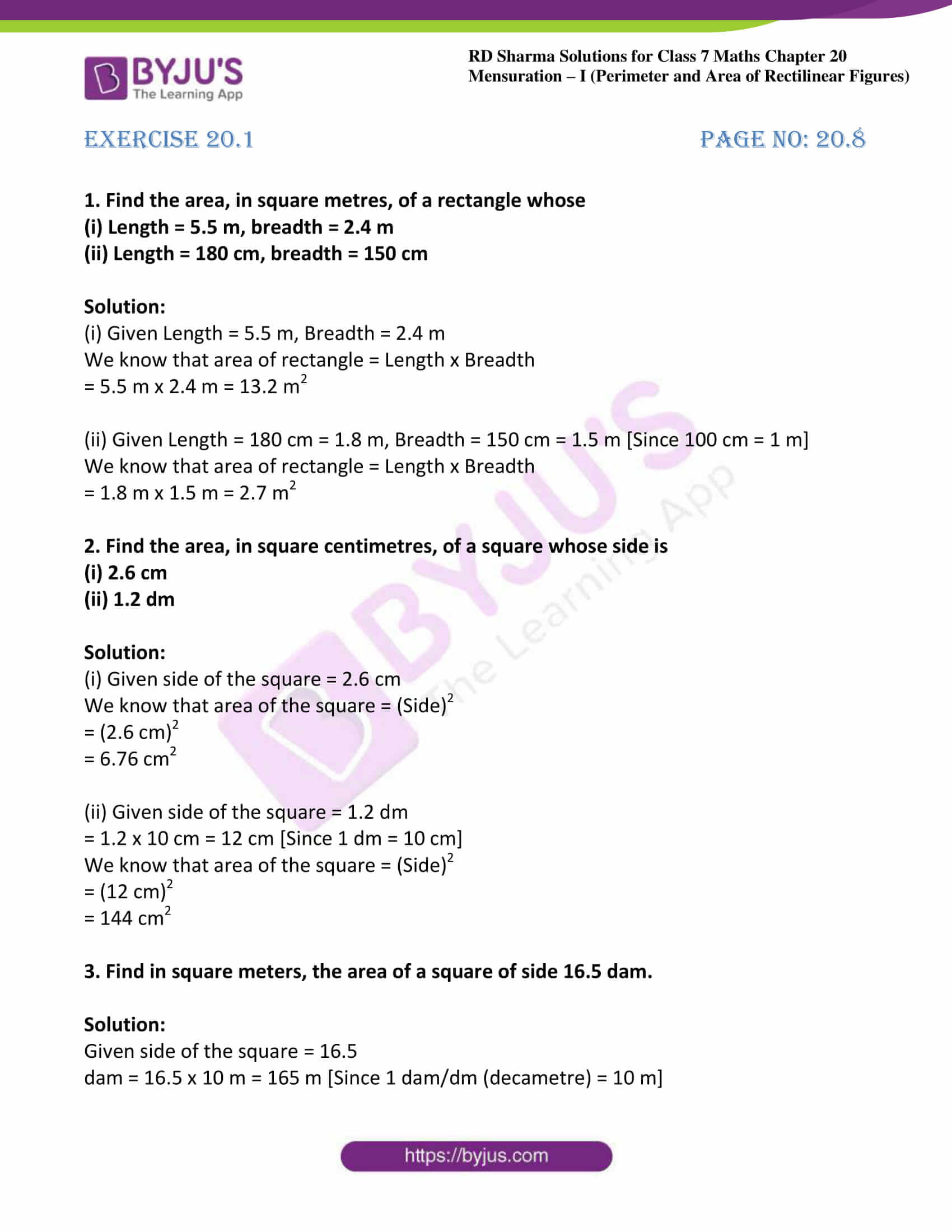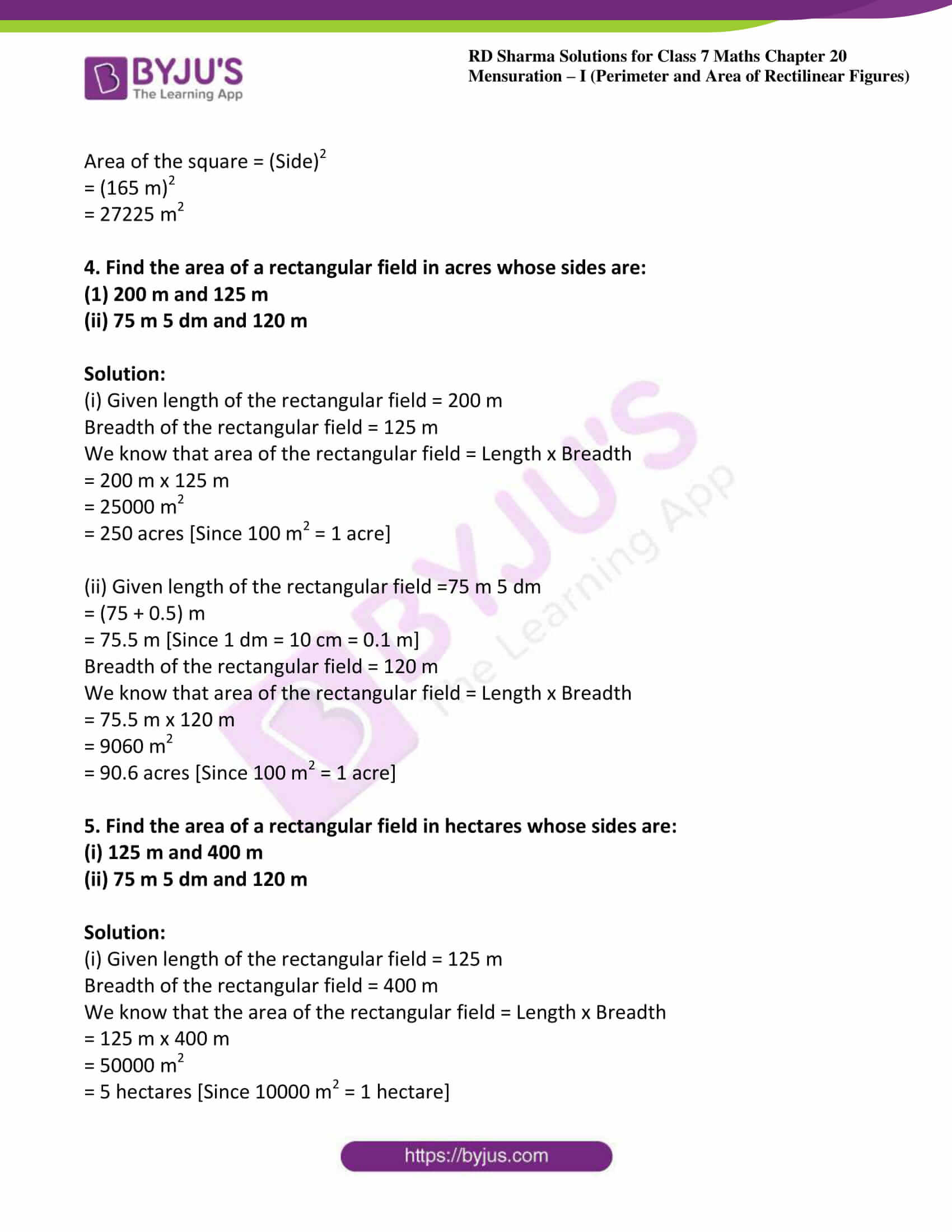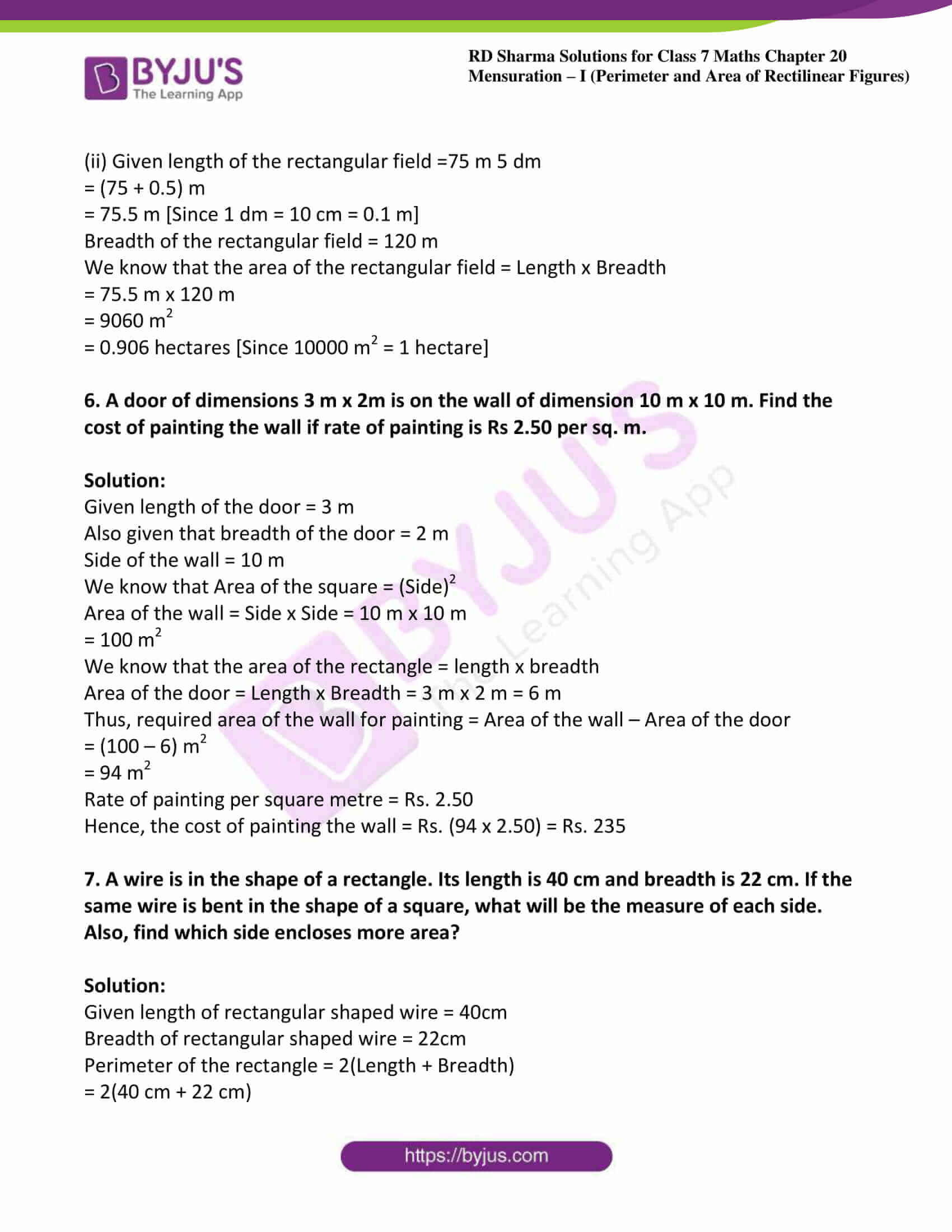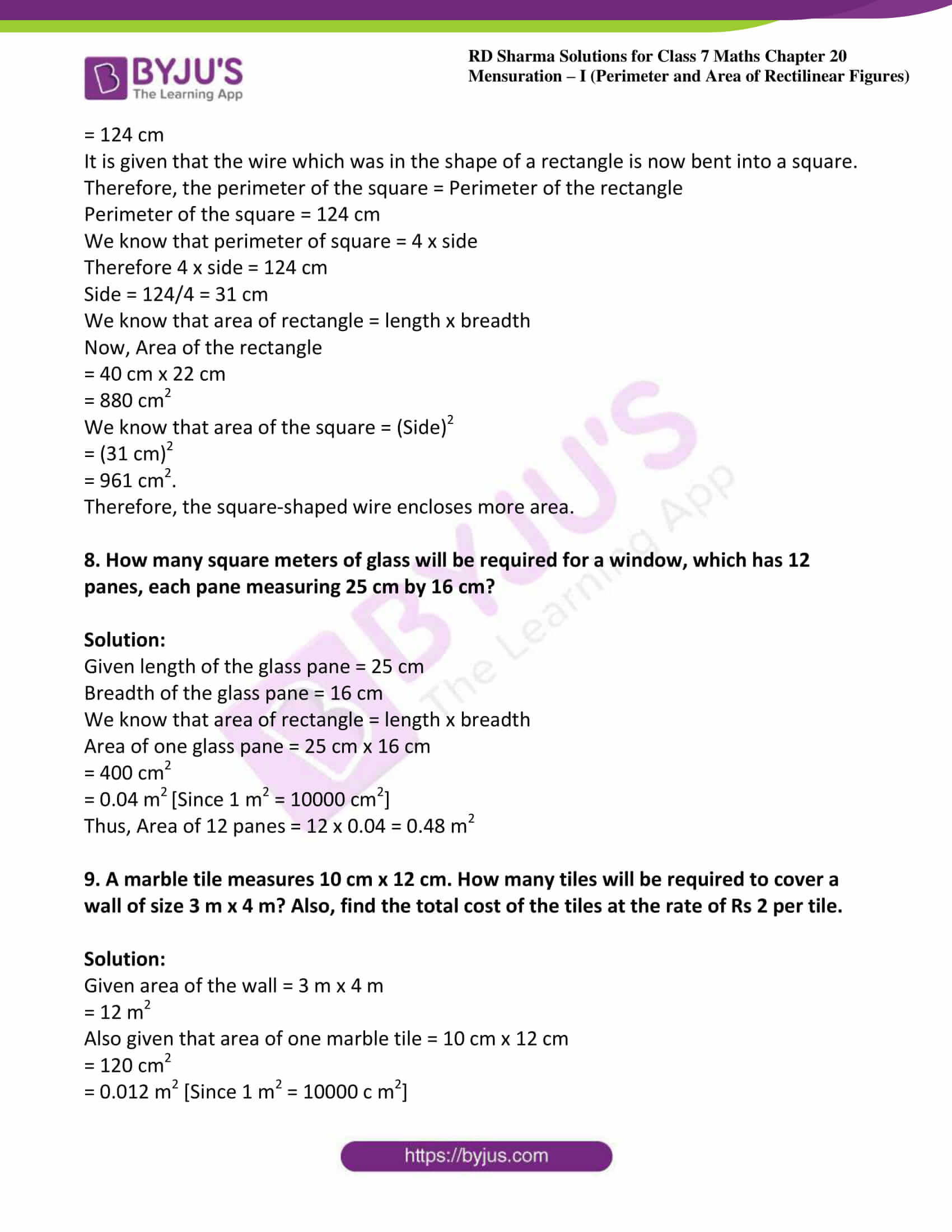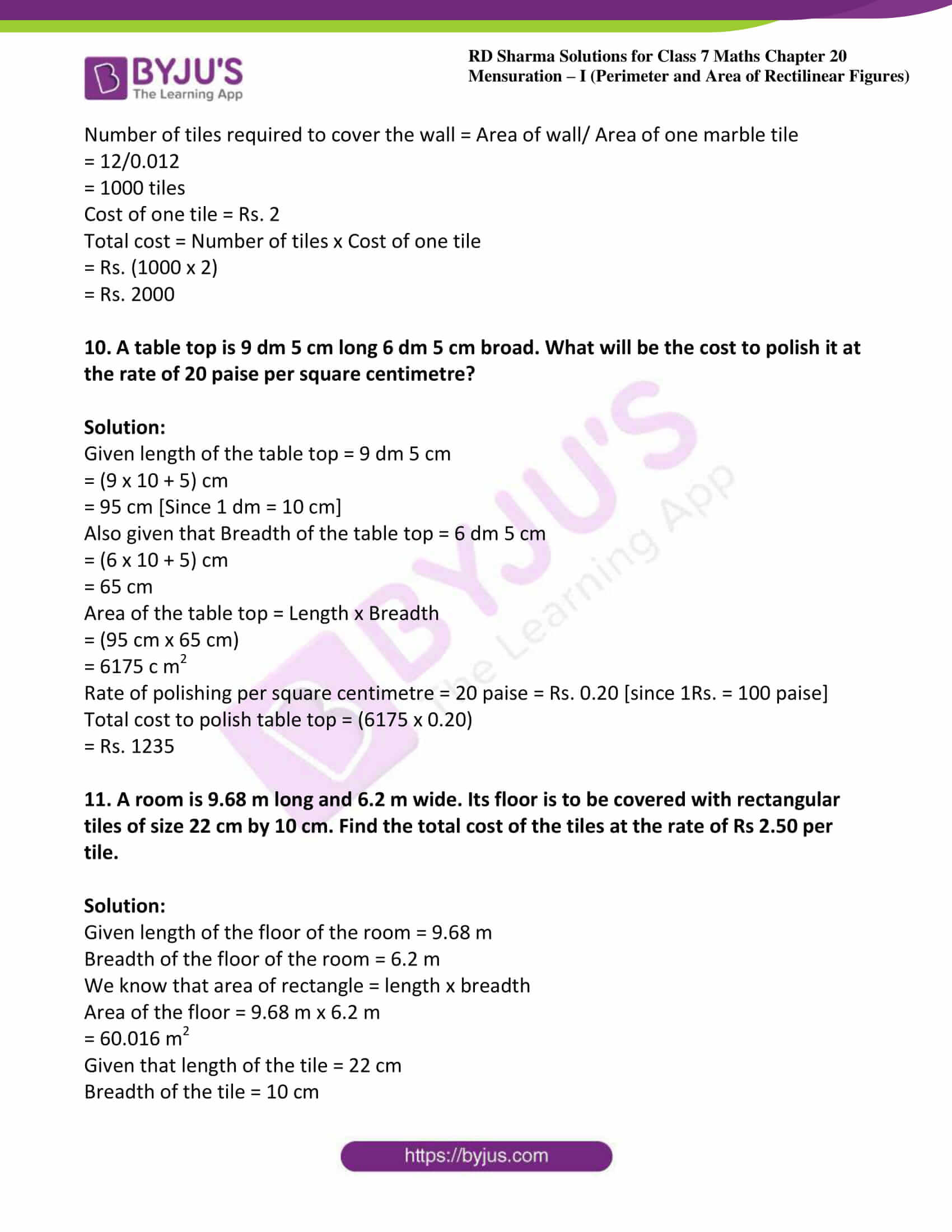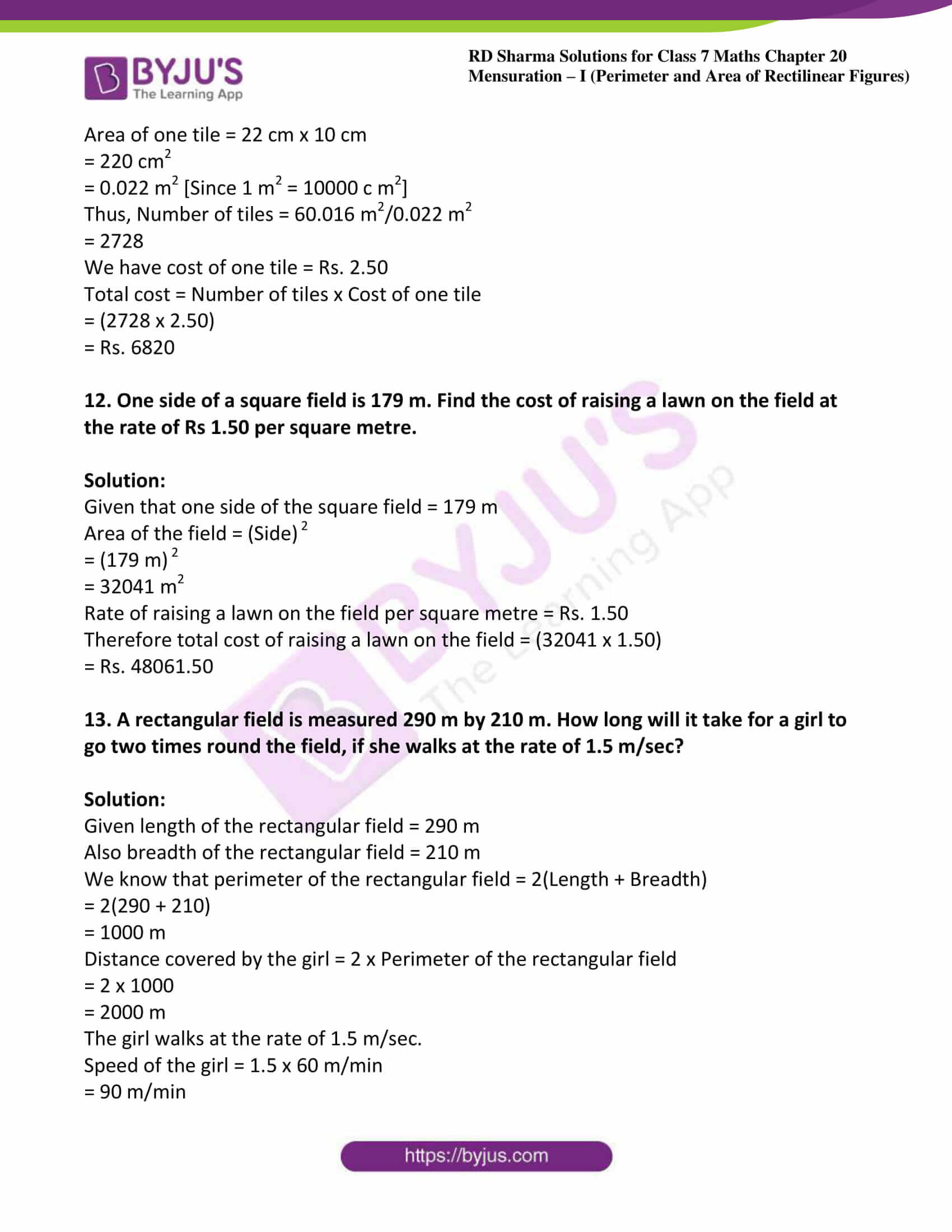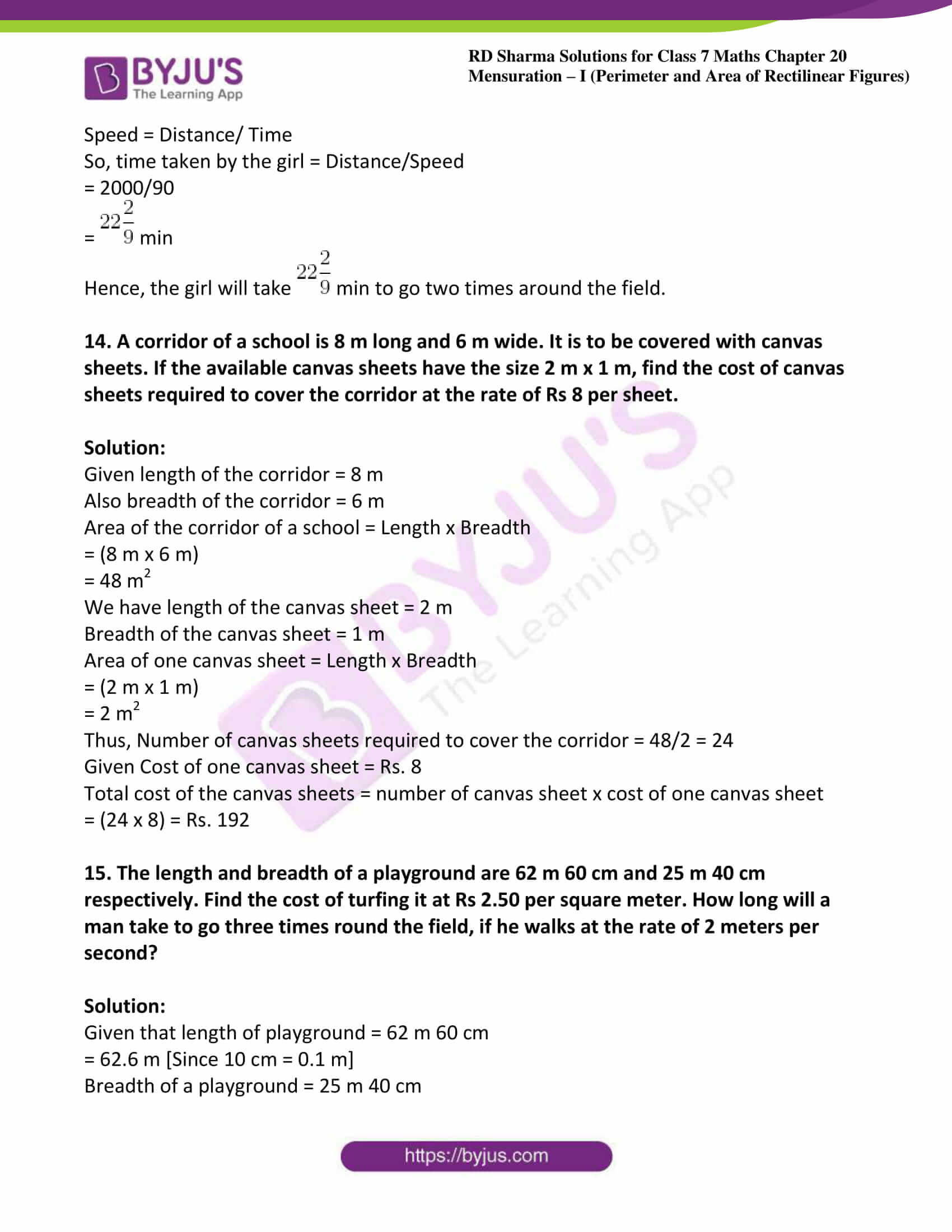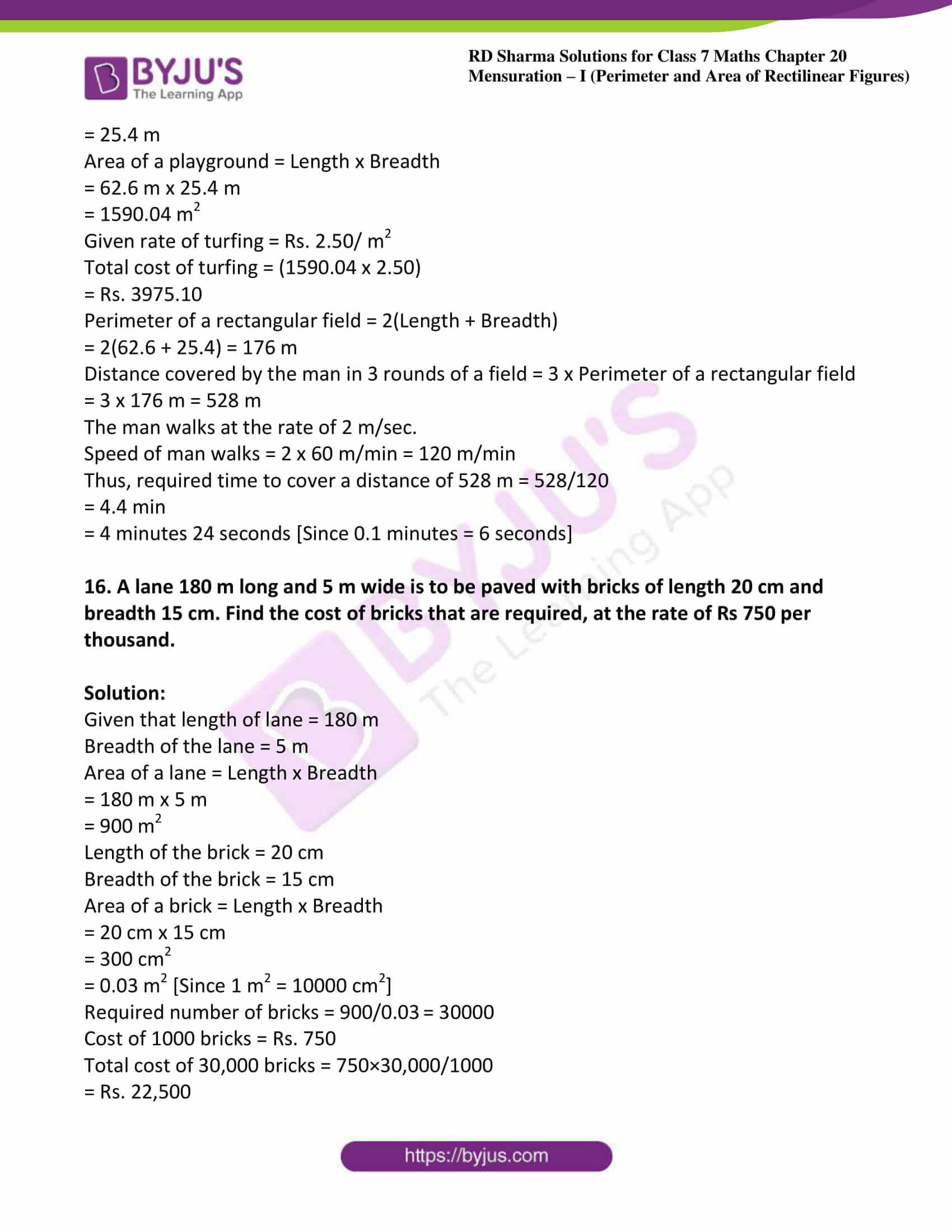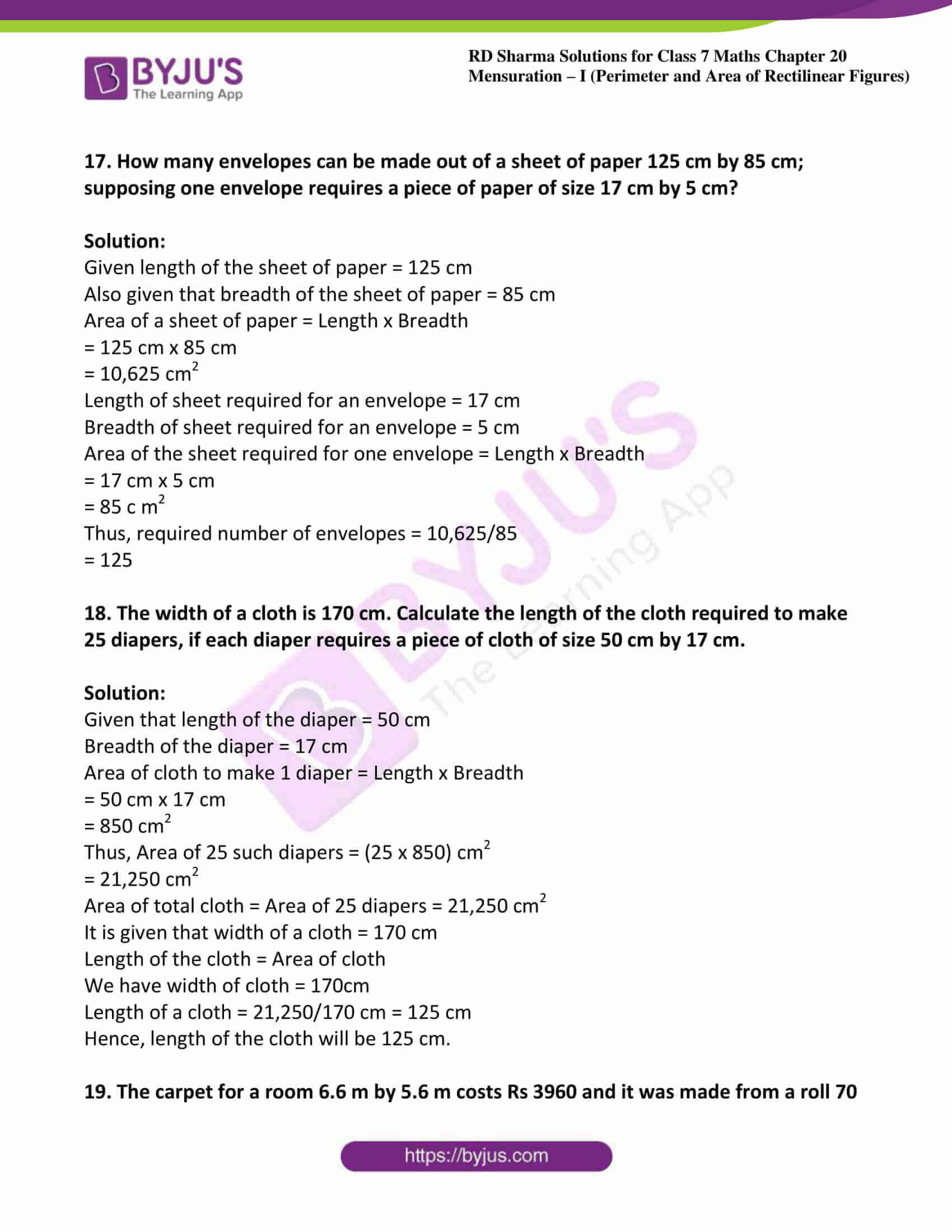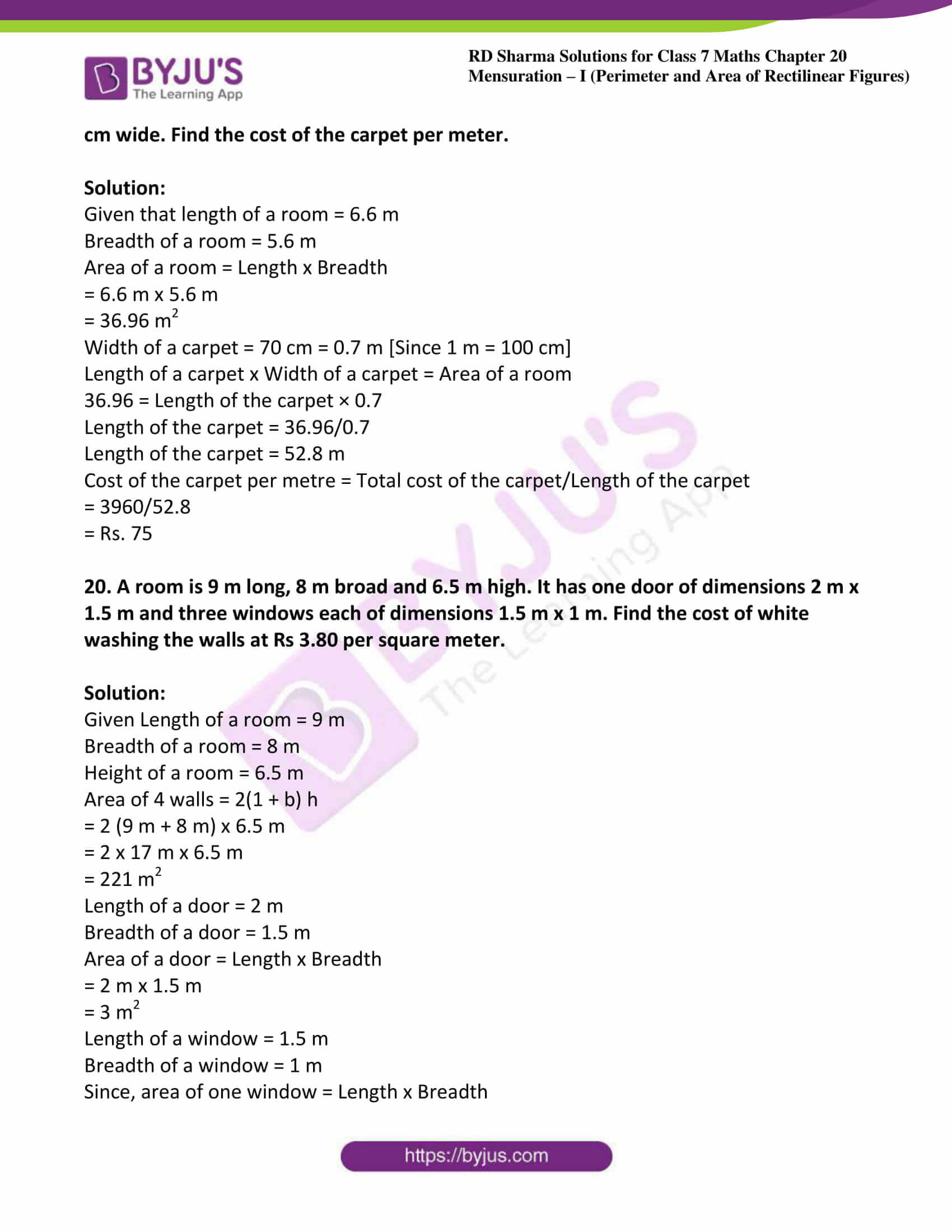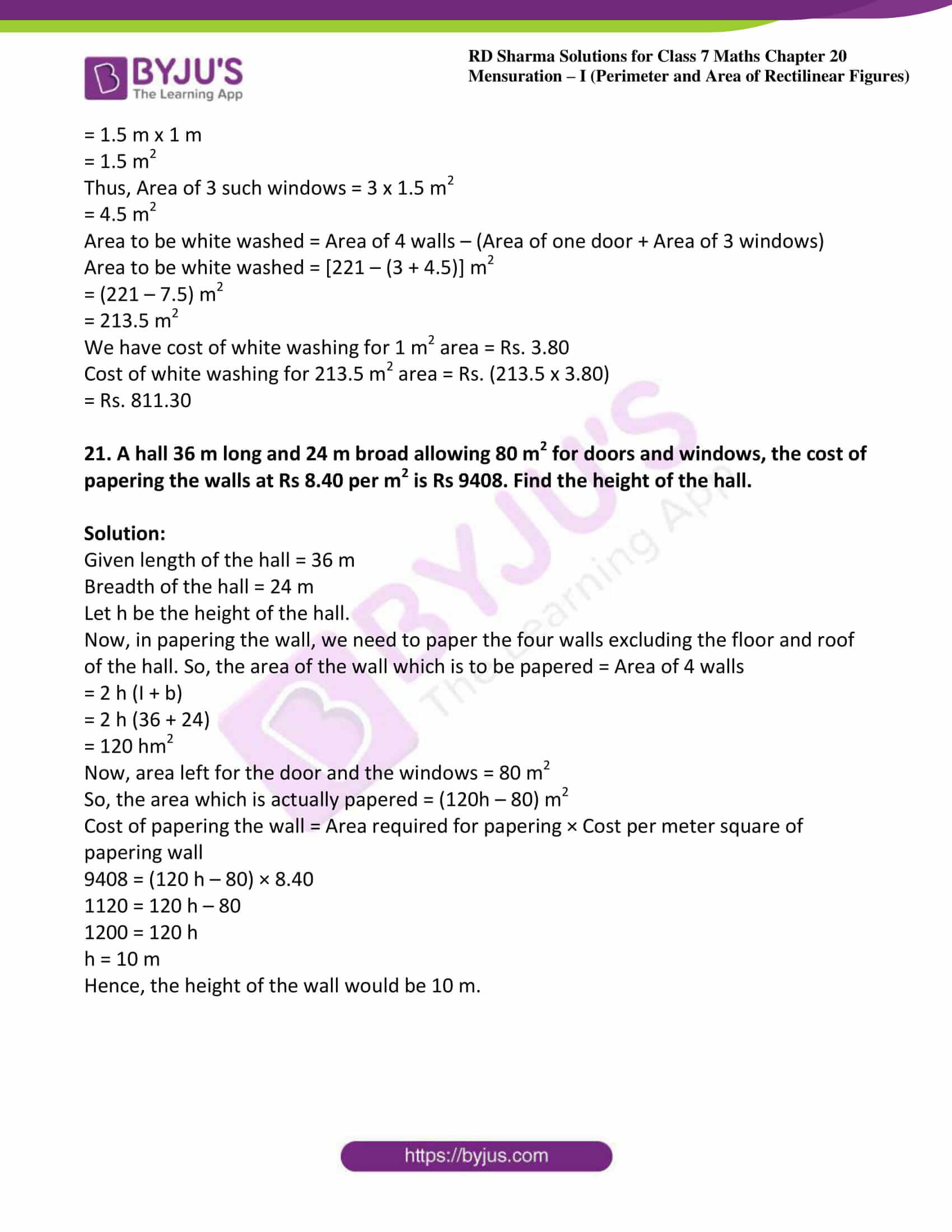### Access answers to Maths RD Sharma Solutions For Class 7 Chapter 20 – Mensuration – I (Perimeter and Area of Rectilinear Figures) Exercise 20.1

Exercise 20.1 Page No: 20.8

1. Find the area, in square metres, of a rectangle whose

(i) Length = 5.5 m, breadth = 2.4 m

(ii) Length = 180 cm, breadth = 150 cm

Solution:

(i) Given Length = 5.5 m, Breadth = 2.4 m

We know that area of rectangle = Length x Breadth

= 5.5 m x 2.4 m = 13.2 m2

(ii) Given Length = 180 cm = 1.8 m, Breadth = 150 cm = 1.5 m [Since 100 cm = 1 m]

We know that area of rectangle = Length x Breadth

= 1.8 m x 1.5 m = 2.7 m2

2. Find the area, in square centimetres, of a square whose side is

(i) 2.6 cm

(ii) 1.2 dm

Solution:

(i) Given side of the square = 2.6 cm

We know that area of the square = (Side)2

= (2.6 cm)2

= 6.76 cm2

(ii) Given side of the square = 1.2 dm

= 1.2 x 10 cm = 12 cm [Since 1 dm = 10 cm]

We know that area of the square = (Side)2

= (12 cm)2

= 144 cm2

3. Find in square meters, the area of a square of side 16.5 dam.

Solution:

Given side of the square = 16.5

dam = 16.5 x 10 m = 165 m [Since 1 dam/dm (decametre) = 10 m]

Area of the square = (Side)2

= (165 m)2

= 27225 m2

4. Find the area of a rectangular field in acres whose sides are:

(1) 200 m and 125 m

(ii) 75 m 5 dm and 120 m

Solution:

(i) Given length of the rectangular field = 200 m

Breadth of the rectangular field = 125 m

We know that area of the rectangular field = Length x Breadth

= 200 m x 125 m

= 25000 m2

= 250 acres [Since 100 m2 = 1 acre]

(ii) Given length of the rectangular field =75 m 5 dm

= (75 + 0.5) m

= 75.5 m [Since 1 dm = 10 cm = 0.1 m]

Breadth of the rectangular field = 120 m

We know that area of the rectangular field = Length x Breadth

= 75.5 m x 120 m

= 9060 m2

= 90.6 acres [Since 100 m2 = 1 acre]

5. Find the area of a rectangular field in hectares whose sides are:

(i) 125 m and 400 m

(ii) 75 m 5 dm and 120 m

Solution:

(i) Given length of the rectangular field = 125 m

Breadth of the rectangular field = 400 m

We know that the area of the rectangular field = Length x Breadth

= 125 m x 400 m

= 50000 m2

= 5 hectares [Since 10000 m2 = 1 hectare]

(ii) Given length of the rectangular field =75 m 5 dm

= (75 + 0.5) m

= 75.5 m [Since 1 dm = 10 cm = 0.1 m]

Breadth of the rectangular field = 120 m

We know that the area of the rectangular field = Length x Breadth

= 75.5 m x 120 m

= 9060 m2

= 0.906 hectares [Since 10000 m2 = 1 hectare]

6. A door of dimensions 3 m x 2m is on the wall of dimension 10 m x 10 m. Find the cost of painting the wall if rate of painting is Rs 2.50 per sq. m.

Solution:

Given length of the door = 3 m

Also given that breadth of the door = 2 m

Side of the wall = 10 m

We know that Area of the square = (Side)2

Area of the wall = Side x Side = 10 m x 10 m

= 100 m2

We know that the area of the rectangle = length x breadth

Area of the door = Length x Breadth = 3 m x 2 m = 6 m

Thus, required area of the wall for painting = Area of the wall – Area of the door

= (100 – 6) m2

= 94 m2

Rate of painting per square metre = Rs. 2.50

Hence, the cost of painting the wall = Rs. (94 x 2.50) = Rs. 235

7. A wire is in the shape of a rectangle. Its length is 40 cm and breadth is 22 cm. If the same wire is bent in the shape of a square, what will be the measure of each side. Also, find which side encloses more area?

Solution:

Given length of rectangular shaped wire = 40cm

Breadth of rectangular shaped wire = 22cm

Perimeter of the rectangle = 2(Length + Breadth)

= 2(40 cm + 22 cm)

= 124 cm

It is given that the wire which was in the shape of a rectangle is now bent into a square.

Therefore, the perimeter of the square = Perimeter of the rectangle

Perimeter of the square = 124 cm

We know that perimeter of square = 4 x side

Therefore 4 x side = 124 cm

Side = 124/4 = 31 cm

We know that area of rectangle = length x breadth

Now, Area of the rectangle

= 40 cm x 22 cm

= 880 cm2

We know that area of the square = (Side)2

= (31 cm)2

= 961 cm2.

Therefore, the square-shaped wire encloses more area.

8. How many square meters of glass will be required for a window, which has 12 panes, each pane measuring 25 cm by 16 cm?

Solution:

Given length of the glass pane = 25 cm

Breadth of the glass pane = 16 cm

We know that area of rectangle = length x breadth

Area of one glass pane = 25 cm x 16 cm

= 400 cm2

= 0.04 m2 [Since 1 m2 = 10000 cm2]

Thus, Area of 12 panes = 12 x 0.04 = 0.48 m2

9. A marble tile measures 10 cm x 12 cm. How many tiles will be required to cover a wall of size 3 m x 4 m? Also, find the total cost of the tiles at the rate of Rs 2 per tile.

Solution:

Given area of the wall = 3 m x 4 m

= 12 m2

Also given that area of one marble tile = 10 cm x 12 cm

= 120 cm2

= 0.012 m2 [Since 1 m2 = 10000 c m2]

Number of tiles required to cover the wall = Area of wall/ Area of one marble tile

= 12/0.012

= 1000 tiles

Cost of one tile = Rs. 2

Total cost = Number of tiles x Cost of one tile

= Rs. (1000 x 2)

= Rs. 2000

10. A table top is 9 dm 5 cm long 6 dm 5 cm broad. What will be the cost to polish it at the rate of 20 paise per square centimetre?

Solution:

Given length of the table top = 9 dm 5 cm

= (9 x 10 + 5) cm

= 95 cm [Since 1 dm = 10 cm]

Also given that Breadth of the table top = 6 dm 5 cm

= (6 x 10 + 5) cm

= 65 cm

Area of the table top = Length x Breadth

= (95 cm x 65 cm)

= 6175 c m2

Rate of polishing per square centimetre = 20 paise = Rs. 0.20 [since 1Rs. = 100 paise]

Total cost to polish table top = (6175 x 0.20)

= Rs. 1235

11. A room is 9.68 m long and 6.2 m wide. Its floor is to be covered with rectangular tiles of size 22 cm by 10 cm. Find the total cost of the tiles at the rate of Rs 2.50 per tile.

Solution:

Given length of the floor of the room = 9.68 m

Breadth of the floor of the room = 6.2 m

We know that area of rectangle = length x breadth

Area of the floor = 9.68 m x 6.2 m

= 60.016 m2

Given that length of the tile = 22 cm

Breadth of the tile = 10 cm

Area of one tile = 22 cm x 10 cm

= 220 cm2

= 0.022 m2 [Since 1 m2 = 10000 c m2]

Thus, Number of tiles = 60.016 m2/0.022 m2

= 2728

We have cost of one tile = Rs. 2.50

Total cost = Number of tiles x Cost of one tile

= (2728 x 2.50)

= Rs. 6820

12. One side of a square field is 179 m. Find the cost of raising a lawn on the field at the rate of Rs 1.50 per square metre.

Solution:

Given that one side of the square field = 179 m

Area of the field = (Side) 2

= (179 m) 2

= 32041 m2

Rate of raising a lawn on the field per square metre = Rs. 1.50

Therefore total cost of raising a lawn on the field = (32041 x 1.50)

= Rs. 48061.50

13. A rectangular field is measured 290 m by 210 m. How long will it take for a girl to go two times round the field, if she walks at the rate of 1.5 m/sec?

Solution:

Given length of the rectangular field = 290 m

Also breadth of the rectangular field = 210 m

We know that perimeter of the rectangular field = 2(Length + Breadth)

= 2(290 + 210)

= 1000 m

Distance covered by the girl = 2 x Perimeter of the rectangular field

= 2 x 1000

= 2000 m

The girl walks at the rate of 1.5 m/sec.

Speed of the girl = 1.5 x 60 m/min

= 90 m/min

Speed = Distance/ Time

So, time taken by the girl = Distance/Speed

= 2000/90

=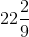min

Hence, the girl will takemin to go two times around the field.

14. A corridor of a school is 8 m long and 6 m wide. It is to be covered with canvas sheets. If the available canvas sheets have the size 2 m x 1 m, find the cost of canvas sheets required to cover the corridor at the rate of Rs 8 per sheet.

Solution:

Given length of the corridor = 8 m

Also breadth of the corridor = 6 m

Area of the corridor of a school = Length x Breadth

= (8 m x 6 m)

= 48 m2

We have length of the canvas sheet = 2 m

Breadth of the canvas sheet = 1 m

Area of one canvas sheet = Length x Breadth

= (2 m x 1 m)

= 2 m2

Thus, Number of canvas sheets required to cover the corridor = 48/2 = 24

Given Cost of one canvas sheet = Rs. 8

Total cost of the canvas sheets = number of canvas sheet x cost of one canvas sheet

= (24 x 8) = Rs. 192

15. The length and breadth of a playground are 62 m 60 cm and 25 m 40 cm respectively. Find the cost of turfing it at Rs 2.50 per square meter. How long will a man take to go three times round the field, if he walks at the rate of 2 meters per second?

Solution:

Given that length of playground = 62 m 60 cm

= 62.6 m [Since 10 cm = 0.1 m]

Breadth of a playground = 25 m 40 cm

= 25.4 m

Area of a playground = Length x Breadth

= 62.6 m x 25.4 m

= 1590.04 m2

Given rate of turfing = Rs. 2.50/ m2

Total cost of turfing = (1590.04 x 2.50)

= Rs. 3975.10

Perimeter of a rectangular field = 2(Length + Breadth)

= 2(62.6 + 25.4) = 176 m

Distance covered by the man in 3 rounds of a field = 3 x Perimeter of a rectangular field

= 3 x 176 m = 528 m

The man walks at the rate of 2 m/sec.

Speed of man walks = 2 x 60 m/min = 120 m/min

Thus, required time to cover a distance of 528 m = 528/120

= 4.4 min

= 4 minutes 24 seconds [Since 0.1 minutes = 6 seconds]

16. A lane 180 m long and 5 m wide is to be paved with bricks of length 20 cm and breadth 15 cm. Find the cost of bricks that are required, at the rate of Rs 750 per thousand.

Solution:

Given that length of lane = 180 m

Breadth of the lane = 5 m

Area of a lane = Length x Breadth

= 180 m x 5 m

= 900 m2

Length of the brick = 20 cm

Breadth of the brick = 15 cm

Area of a brick = Length x Breadth

= 20 cm x 15 cm

= 300 cm2

= 0.03 m2 [Since 1 m2 = 10000 cm2]

Required number of bricks = 900/0.03 = 30000

Cost of 1000 bricks = Rs. 750

Total cost of 30,000 bricks = 750×30,000/1000

= Rs. 22,500

17. How many envelopes can be made out of a sheet of paper 125 cm by 85 cm; supposing one envelope requires a piece of paper of size 17 cm by 5 cm?

Solution:

Given length of the sheet of paper = 125 cm

Also given that breadth of the sheet of paper = 85 cm

Area of a sheet of paper = Length x Breadth

= 125 cm x 85 cm

= 10,625 cm2

Length of sheet required for an envelope = 17 cm

Breadth of sheet required for an envelope = 5 cm

Area of the sheet required for one envelope = Length x Breadth

= 17 cm x 5 cm

= 85 c m2

Thus, required number of envelopes = 10,625/85

= 125

18. The width of a cloth is 170 cm. Calculate the length of the cloth required to make 25 diapers, if each diaper requires a piece of cloth of size 50 cm by 17 cm.

Solution:

Given that length of the diaper = 50 cm

Breadth of the diaper = 17 cm

Area of cloth to make 1 diaper = Length x Breadth

= 50 cm x 17 cm

= 850 cm2

Thus, Area of 25 such diapers = (25 x 850) cm2

= 21,250 cm2

Area of total cloth = Area of 25 diapers = 21,250 cm2

It is given that width of a cloth = 170 cm

Length of the cloth = Area of cloth

We have width of cloth = 170cm

Length of a cloth = 21,250/170 cm = 125 cm

Hence, length of the cloth will be 125 cm.

19. The carpet for a room 6.6 m by 5.6 m costs Rs 3960 and it was made from a roll 70 cm wide. Find the cost of the carpet per meter.

Solution:

Given that length of a room = 6.6 m

Breadth of a room = 5.6 m

Area of a room = Length x Breadth

= 6.6 m x 5.6 m

= 36.96 m2

Width of a carpet = 70 cm = 0.7 m [Since 1 m = 100 cm]

Length of a carpet x Width of a carpet = Area of a room

36.96 = Length of the carpet × 0.7

Length of the carpet = 36.96/0.7

Length of the carpet = 52.8 m

Cost of the carpet per metre = Total cost of the carpet/Length of the carpet

= 3960/52.8

= Rs. 75

20. A room is 9 m long, 8 m broad and 6.5 m high. It has one door of dimensions 2 m x 1.5 m and three windows each of dimensions 1.5 m x 1 m. Find the cost of white washing the walls at Rs 3.80 per square meter.

Solution:

Given Length of a room = 9 m

Breadth of a room = 8 m

Height of a room = 6.5 m

Area of 4 walls = 2(1 + b) h

= 2 (9 m + 8 m) x 6.5 m

= 2 x 17 m x 6.5 m

= 221 m2

Length of a door = 2 m

Breadth of a door = 1.5 m

Area of a door = Length x Breadth

= 2 m x 1.5 m

= 3 m2

Length of a window = 1.5 m

Breadth of a window = 1 m

Since, area of one window = Length x Breadth

= 1.5 m x 1 m

= 1.5 m2

Thus, Area of 3 such windows = 3 x 1.5 m2

= 4.5 m2

Area to be white washed = Area of 4 walls – (Area of one door + Area of 3 windows)

Area to be white washed = [221 – (3 + 4.5)] m2

= (221 – 7.5) m2

= 213.5 m2

We have cost of white washing for 1 m2 area = Rs. 3.80

Cost of white washing for 213.5 m2 area = Rs. (213.5 x 3.80)

= Rs. 811.30

21. A hall 36 m long and 24 m broad allowing 80 m2 for doors and windows, the cost of papering the walls at Rs 8.40 per m2 is Rs 9408. Find the height of the hall.

Solution:

Given length of the hall = 36 m

Breadth of the hall = 24 m

Let h be the height of the hall.

Now, in papering the wall, we need to paper the four walls excluding the floor and roof of the hall. So, the area of the wall which is to be papered = Area of 4 walls

= 2 h (I + b)

= 2 h (36 + 24)

= 120 hm2

Now, area left for the door and the windows = 80 m2

So, the area which is actually papered = (120h – 80) m2

Cost of papering the wall = Area required for papering × Cost per meter square of papering wall

9408 = (120 h – 80) × 8.40

1120 = 120 h – 80

1200 = 120 h

h = 10 m

Hence, the height of the wall would be 10 m.

#### 1 Comment

1. Very nice explanations